3D Geometry Primer: Chapter 2 - Issue 03 - Planes and Parametric Equations by (13 September 2002) Return to The Archives
 IntroductionYou know what? The hardest part of an issue to write is the introduction, and since I can't think of anything, I'll go for the easiest solution and write how difficult it is to write the introduction of an issue. Then I could tell you that the easiest way out is to write how hard it is to write a proper intro. And before I end up in a vicious circle (I'm afraid it's already too late though), I'll say that today we'll start with the topic of planes. Now lets start all over again..."Chocolate is heavenly, mellow, sensual, deep. Dark, sumptuous, gratifying, potent, dense, creamy, seductive, suggestive, rich, excessive, silky, smooth, luxurious, celestial ... Chocolate is downfall, happiness, pleasure, love, ecstasy, fantasy ... Chocolate makes us wicked, guilty, sinful, healthy, chic, happy.", Elaine ShermanA plane is an infinitely large flat area in 3D space. If you walk on a plane, you can go in any direction you like, you will never reach the boundaries of that plane; simply because there are none.It is impossible to represent infinitely large planes on screen, so I'll always show a little "plate" of that plane. So, if you see it, know that it is in fact a real plane. See the difference between the image of a plane and a polygon. On the left you can see a plane which has no boundaries, on the right you can see a square which is bounded by 4 edges.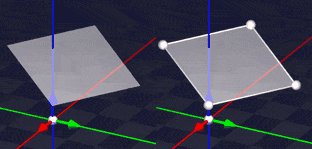(I) Parametric Equation, An Expansion Of The Line?The best way to imagine a parametric equation of a plane is to think about a line d that is moved parallel along another line e. Indeed, if we move d from one end of e to the other end, we'll have described a surface which is as flat and as infinitely large as a plane. But what is it mathematically?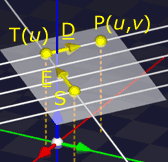Imagine a line d with a starting point T and direction vector D. It's parametric equation is: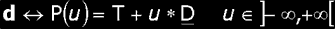Nothing special about that, except maybe for the weird choice of some letters and the explicit mentioning of the fact that the point P is indeed a function with parameter u.Apply one trick now: make the start point T dependent on an extra parameter v:This implies that the support point of the line d can be changed by changing the value v, and hence, the line will be changed too. That's why we also write the parameter v next to the letter d of the line: to indicate that you will have another line if you fill in another v.Notice also that now the point P depends on 2 variables u and v: the first one indicates the position of the point on the line, the second indicates the line itself. By changing the value v we change the line d. But in what way? Notice that we don't vary the direction vector D, hence all these lines will have the same direction and be parallel to each other.Apply a second trick now: say that the function for our support point T(v) is nothing more than a point of another line e: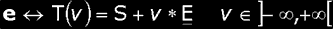If we vary v from –Ą to +Ą, we'll move d along that line e. Hence, d(v) will describe our desired plane: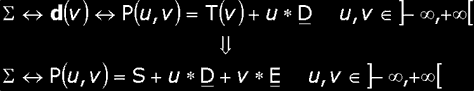Notice that we use a "greek capital" S to indicate a plane here. As you can see, the parametric equation of a plane has one support point S(s1, s2, s3) and two direction vectors D(d1, d2, d3) and E(e1, e2, e3). For these two direction vectors, it takes two parameters u and v. This is why we say a plane is a 2D object: it takes 2 parameters to identify 1 point of the object. Other notations of the *same* parametric equation are: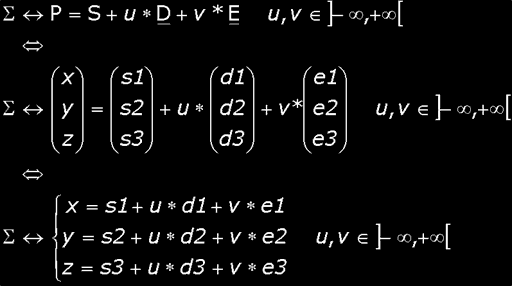Remark: Convince yourself that d and e can *not* be parallel. If they do so, then they will be automatically coincident (= be the same line), since they share at least point T(v). Hence, you would end up moving d along itself. You can imagine that you would never describe a plane that way. If you don't understand that, check out issue II.2, paragraph (ii) on relations between lines.An Example: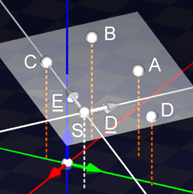A = P(2, 1); B = P(5/2, 3/2); C = P(0, 2); D = P(-2/3, 3/2)An Exercise: You have a plane S <-> P(u, v) = S + v * E + u * D with S(7, –1, 3), D(2, 8, –6) and E(–1, –4, 3). Find the coordinates of the points P(2, 3) and P(5, 9)? What do you notice and how can you explain it?To have a 2D object like a plane, there may only correspond *one* (u, v) pair with each point of the object. If this is not the case, like in the exercise, you will not have a plane, but a line. And not a very good line! A line is a 1D object and takes only one parameter. Here you will use two, and every point of the line will correspond with infinitely many (u, v) pairs.Exercise: Try to find other (u, v) pairs for that same point of the previous exercise. For each value of u you think of, you will be able to find another v. Can you find a function v(u) that returns the corresponding v for each u? Try to find a function u(v) too. Notice that this function depends on that particular point. If you take another point, e.g. (5, –9, 9), then you will find other functions. Try that too!You can imagine that this is not a very good thing! So, always be aware that D and E can *never* be parallel.

 (II) You'd Better Keep The Direction Vectors OrthonormalAm I wrong if I say you only succeeded in finding u and v by trial and error? Probably not. That's because we have ugly direction vectors. Now I'll teach you how to find nice ones. The trick is in the dot product...First off, let me tell you what we really want. We want to find u and v so that: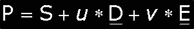Furthermore, we want to find these values by two simple dot products: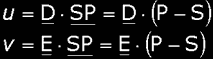If you try this on the data I gave you, you'll notice that it doesn't work out. You get the wrong parameters. Therefore, we'll examine what conditions must be met to use these equations. I'll do this by taking a general point P = S + u * D + v * E and using that point in these equations: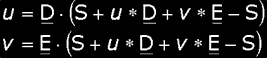After some rearranging, you'll find the following: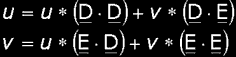Since you definitely want u = u and v = v, you can easily see that next conditions need to be fulfilled to be true: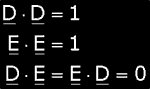In plain english, this mean that both D and E must be normalized and that they must be orthogonal to each other. In one word: orthonormal. "That's all very nice, but what should I do if I don't have orthonormal direction vectors?", you ask. Two options:a) Orthonormalize ThemThe easiest way is to create new direction vectors for your plane that *are* orthonormal. This can done with the usual Gramm-Schmidt procedure: OrthogonalizingNormalizing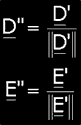Now, use D" and E" as new direction vectors.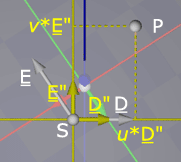b) Use reciprocal direction vectorsSometimes you simple can't use other direction vectors. It may happen that you simply cannot change the current direction vectors because they represent other valuable data too. I'm not going to give an example of this, but if you're in this case, you'll know it. Then you can use this approach. You'd better use (a) if you can.We still want to use a single dot product to get u and v but without orthonormalizing those direction vectors. Therefore we'll invent two new vectors Dr and Er: the reciprocal direction vectors. These vectors will substitute the original direction vector if you want to calculate the parameter values u and v: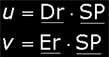Though, you still want to use these parameter values with the original direction vectors! You still want that u and v give you this:To do that, we'll look at what information about v sneaks into the dot product Dr · SP and we'll try to filter that out to get u only.Let's substitute again! With P = S + u * D + v * E we get again:Since we want u = u, we can now see that we want Dr · D = 1 and Dr · E = 0. So we must find a vector Dr orthogonal to E and which dots with D to 1.The first part is easy, we'll create a vector A which is orthogonal to E by using the same approach as in (a). Then, we scale it to get Dr so that Dr · D = 1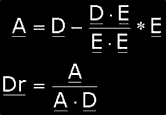Do the same thing for E:The vectors Dr and Er are called the reciprocal basevectors of the the plane. Notice that this approach also works for higher dimensions (with more than two direction annex base vectors).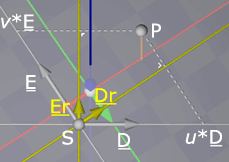Exercise: Try out both solutions with the previous data I gave you.

 (III) Barycentric Parametric Equations, The Forgotten OnesIt's also possible to define a plane by claiming that it goes through 3 different and non-collinear points A, B and C. Try to convince yourself that this is true! If you only have 2 points, you'll be able to create a lot of different planes that go through these points. If you have 4 or more points, you will not always be able to find a plane that goes through all of them! Also, imagine what happens if the three points ARE collinear (= lie on 1 straight line) ...a) The First OneI have already discussed barycentric combinations in chapter I issue 9. Basically, a parametric equation takes n points of the object and (n – 1) parameters to return the generic point P of the object. In the case of a plane, it takes 3 points A, B and C, and 2 parameters u and v. In paragraph (iv) d), I've proposed the following equation: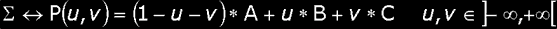Exercise: prove to yourself that this is indeed an equation of the plane through A, B and C. Do this by rewriting this equation in our "standard" parametric equation P(u, v) = S + v * E + u * D. i.e. tell me what S, D and E look like, by using the points A, B and C. Also prove that these points are indeed on the plane! Do this by finding proper u and v values for the three different points.b) The Second OneThere's also another barycentric equation for that same plane through A, B and C. This one is a bit tricky. If you don't understand it, you may skip it and go straight to paragraph (iii) with a clear conscience :)Notice that I've used another letter for the generic point here: Q(u, v). This is because for the same (u, v) pair, both equations will give you different points of the plane. However both equations still give points of the *same* plane, hence we can call both planes with the same name: S.A problem with this second equation, is that it is *not* linear in u and v! The reason is that there's a term in the equation with factor u * v. If you fully expand the equation, you can clearly see this: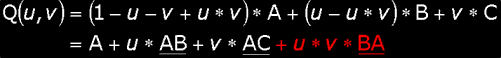You can ask: is it still a plane? The answer is trivial: yes. But why? Maybe the equation isn't linear in u and v anymore, but it's linear in something else. We'll have to transform them into new parameters. Consider the following:Now substitute this into the equation, and compare it to the first barycentric equation ... You see it? It only differs with equation (a) by the accents. It's linear again. Well, that's about everything there is to say about it. Keep an eye on the accents however! In the second equation, you feed u and v, but you use u' and v'. Be careful about that!c) The Difference, And An ExerciseMaybe one more thing ... What happens if you feed in all values for u and v between 0 and 1? Feed in the following pairs for (u, v), and check out what points are returned.These four pairs are the vertices of the 2D-interval [0, 1] × [0, 1]. Each other (u, v) pair in that interval will be mapped to a point between them. For the first equation you'll get a filled quadrilateral, for the second a filled triangle.This is all about parametric equations for planes now. I hope you enjoyed it and learned a few new things. Next week we'll apply the "Super Trick"!Thank you Nick,Tot de volgende keer maar weer, Brammetje Bram
 Article Series:3D Geometry Primer: Chapter 2 - Issue 01 - Appendix3D Geometry Primer: Chapter 2 - Issue 01 - Points And Lines3D Geometry Primer: Chapter 2 - Issue 02 - Appendix3D Geometry Primer: Chapter 2 - Issue 02 - More About Lines3D Geometry Primer: Chapter 2 - Issue 03 - Planes and Parametric Equations3D Geometry Primer: Chapter 2 - Issue 04 - Cartesian Equations and Normal Vectors